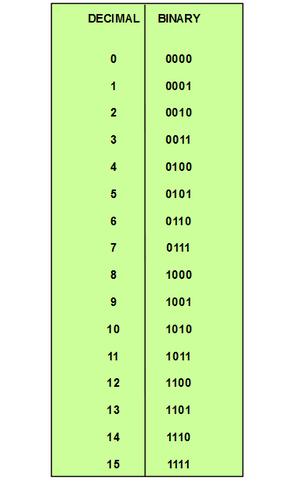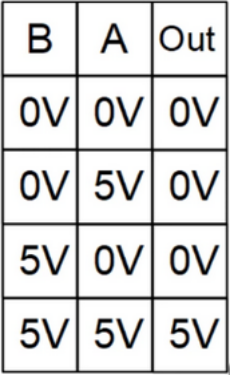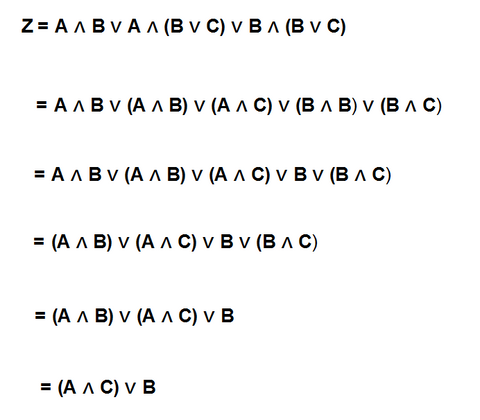# Introduction

Computers think in a kinda funny way. They think binary so the only thing on their mind are 0’s and 1’s. In the context of digital circuits, binary refers to a system of representing information or data using only two possible values or states. These values are typically represented as 0 and 1, which correspond to the states of "off" and "on" in a digital circuit.

Digital circuits, such as those found in microcontrollers, use binary signals to communicate and process data. The binary signals are created by manipulating the voltage levels of the electrical signals that flow through the circuit.

For example, a microcontroller might use a binary signal to control an LED. A binary value of 1 might be used to turn the LED on, while a binary value of 0 would be used to turn it off.

## Binary

Binary is also used in the representation of numbers in digital circuits. In binary, each digit can only have a value of 0 or 1. So how can one count if we only can use 0 and 1?
Actually counting in binary is similar to counting in decimal, except that binary only has two possible values! Let's continue to learn to count to 15 in binary.

To count to 15 in binary we need 4 places for our numbers 0 and 1.
We start with all places holding a zero [0 0 0 0] - this is equal to 0. Ok, so far so good! If we then flip the rightmost digit to a 1 [0 0 0 1], then we have the number 1 represented in binary.
What about the number 2 then? For number 2 we move our digit 1 one place to the left [0 0 1 0]. Now we have the rightmost place holding a zero, like we did with the binary representation of 0. If we then flip that place to a 1, we count a single digit up - so 3 in binary would be [0 0 1 1]. For the binary representation of 4, we notice that we can’t flip any 0 til a 1 to count up, so we replace the 1’s on the fourth and third place with 0’s and place a 1 in the second place. So 4 in binary looks like this [0 1 0 0]. (This was also the method we used when we counted from 1 to 2 in binary).
This is the method of counting in binary. Try for yourselves and see if you can understand the binary numbers in the figure 1:Figure 1: shows the decimal numbers and their corresponding binary representation up to the number 15.

You might have already guessed that if we wanted to represent a number larger than 15 we would need more than 4 places to hold the digits. And so this is how a computer counts and basically receives and delivers all information, from the pixels to your screen to the most complex calculations when you play your favorite computer game! Let’s just say that computers are very fast at counting binary numbers.

## Logic Gates

Logic gates are like the basic building blocks of a digital circuit. Like lego we can put them together for various constructions. Some of these gates are called AND, OR, NOT, NAND, NOR, XOR, and XNOR.

All of these gates have two inputs and one output, except for the NOT which only has one input. The NOT gate is also known as an inverter, as it turns 1’s into 0’s and vice versa. The logic gates each have a symbol that represents them in digital circuits, see figure 2:Figure 2: Logic gates. The inputs are represented with the two lines on the left into the gates. The output is the single line to the right of the gates.

The AND gate produces a logic 1 output only when all of its inputs are logic 1.
So whether the output is 0 or 1 of the logic gates depends on the combination of the two inputs for the particular logic gate. Below is an overview of these combinations for the rest of the gates mentioned:

OR gate: This gate produces a logic 1 output when at least one of its inputs is logic 1.

NOT gate: This gate produces the complement of its input; that is, a logic 1 input produces a logic 0 output, and vice versa.

NAND gate: This gate is a combination of an AND gate and a NOT gate. It produces a logic 0 output only when all of its inputs are logic 1.

NOR gate: This gate is a combination of an OR gate and a NOT gate. It produces a logic 1 output only when all of its inputs are logic 0.

XOR gate: This gate produces a logic 1 output when exactly one of its inputs is logic 1.

XNOR gate: This gate is a combination of an XOR gate and a NOT gate. It produces a logic 1 output when both of its inputs are the same.

By assembling these gates in a digital circuit, the gates will make decisions based on a combination of digital signals coming from its inputs. It is wanted to use as few gates as possible, as it makes the circuits more effective and so the calculations require less CPU and are done faster. That's why logic gates are based on Boolean algebra. Boolean algebra is a mathematical method one can use to figure out the most effective construction of gates that is needed to perform as the application requires.

## Boolean algebra

Let's dive a little further into this by exploring the AND gate. In the figure below, figure 3, you’ll see the construction of a circuit of an AND gate.Figure 3Illustrates the circuit of an AND gate.
Voltage sources: Vin, A and B
Transistors: T1 and T2.
Resistors: Ra , Rb and Rout

In the blog about analog circuits we learned that if we connect 5 V to the collector of transistor T1 (Vin) on figure 3 and there's no voltage from A or B there will be zero voltage for the output (Vout). If we change the voltage at A to 5 V there's still no voltage for the output. Same thing for B. Only when both A and B are applied 5 volts will we have 5 volts at the output, because this would mean that both transistors are open.
This concept for the circuit of the AND gate is demonstrated in the figure below.Figure 4: Notice that the only situation where we get the 5 V at the output, is when both A and B have 5 V.

In digital electronics there are only high and low, 1 and 0, logic levels.
If we then replace the 5 V and 0 V with 1’s and 0’s we get what is called truth tables. This concept is the beginning of digital electronics.Figure 5: Truth table for the AND gate.

Truth tables can be described using an equation. All gates have truth tables and their own equations, though the mathematics used in digital electronics is different from regular math and as we mentioned a little earlier it's called boolean algebra.

We can imagine we had a somewhat complex digital ciruit like the one showed below. Here we have three inputs: A, B and C. And one output: ZFigure 6: A digital circuit.
Inputs: A, B and C
Output: Z

This might look very smart and handy, but maybe we can do a little better.
So how do we do that? We use boolean algebra.
We start with writing the boolean expression for the digital circuit in figure 7.

The expression for the digital circuit above is:Figure 7
: Boolean expression for the circuit seen in figure 6.

Then we use boolean algebra methods to reduce this complex expression into its simplest form.Figure 8: Different expressions derived by boolean algebra to desribe the circuit seen in figure 6.

All of the expressions seen right above are equivalent. They might each describe a different construction of a circuit but all of these circuits do exactly the same. This means that the behavior of all these circuits can be described by the same truth table shown below.

 A B C Z 0 0 0 0 0 0 1 0 0 1 0 1 0 1 1 1 1 0 0 0 1 0 1 1 1 1 0 1 1 1 1 1

Figure 9: Truthtable for the circuit in figure 6 and for all expressions seen in figure 8.

So we started with this big complex digital circuit seen in figure 6 and by using boolean algebra we reduced that circuit to one that can be desribed by the same truth table but with only 3 gates.Figure 10: The reduced circuit from figure 6.

This circuit needs less components, so it's more reliable, it's faster, it generates less heat and it is cheaper to make. This is the reason why boolean algebra is an extremely powerful tool for designing digital circuit and computers.

The Laws og Boolean Algebra
In order for us to utilize this extremely helpful tool we need to know about its laws.
This will require some practice as there are quite a few laws to learn.
These laws are:

• Annulmen
• Identity
• Idempotent
• Complement
• Double Negation
• De Morgan's Law
• Associative
• Commutive
• Distributive
• Absorptive

If you feel ready to go even further and broaden your understanding of digital circuits by learning more about boolean algebra, this place is a great way to get started. Everything is explained from the bottom and then a few examples of boolean algebra is showcased.
The actions performed by digital circuits are often remembered by the computer in what is called memory elements and registers.

Memory Elements and Registers
These are circuits that store binary information, such as RAM, ROM, and EPROM. You can picture it as a library that categorizes and stores information into their specific sections, so the information can be found and used easily. To create these registers requires a good understand of coding and will also require some effort to learn just like boolean algebra. But why do we need to store this data in the first place. Well theres a ton of different reasons - maybe we want to operate, compare or share the information in our memory elements and registers. This is done by sequential circuits.

### Sequential circuits

Sequential circuits are digital circuits that use memory elements and registers to store information and operate on that information in a specific sequence, based on a clock signal. It’s how a computer plans its calculations by a certain hierarchy of actions, so it functions in the most effective way to produce its purpose. These circuits can be categorized into two types: Synchronous Sequential Circuits and Asynchronous Sequential Circuits.

Synchronous Sequential Circuits
In synchronous sequential circuits, the memory elements are connected in a way that the output of one memory element feeds into the input of another memory element, forming a chain of feedback. These circuits operate in synchronization with a clock signal, which ensures that the signals are sampled and stored at the same time. The state of the circuit at any given time is determined by the current input and the previous state..

Asynchronous Sequential Circuits
In asynchronous sequential circuits, the memory elements are connected in a way that the output of one memory element feeds into the input of another memory element without any feedback, forming a combinatorial logic circuit. These circuits do not rely on a clock signal and the output of the circuit can change at any time based on the inputs. The state of the circuit at any given time is determined by the inputs and the previous state.

Arithmetic Circuits
These circuits perform arithmetic operations, such as addition, subtraction, multiplication, and division.

### Data Converters

These are circuits that convert digital signals into analog signals and vice versa, such as ADCs and DACs. ADCs and DACs are very useful for connecting the real analog world to the digital world. You can think of them as translators between the two.
Have you ever wondered how a thermostat knows and shows the correct temperature? Well, it does so by measuring the analog temperature by a sensor, and then it maps that value into its calculation to display the correct temperature digitally.

Skriv kommentar!